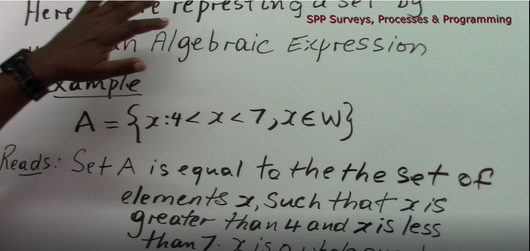Set Builder Notation

Regular price \$2.00 Sale

This 12:21 minute (575MB) Math Tutorial on Set Builder Notation is done by M. Chan (Trained Certified Teacher).

Examples worked in this tutorial include:

• Set Builder Notation Introduction
• Symbols Used (Natural Numbers, Whole Numbers, Integers and Rational Numbers)
• Example worked A={x: 4 < x < 7, x Ꜫ W}
• B={x: -3 ≤ x ≤ 8, x Ꜫ Z}
• C={x: 8 ≤ x < 13, x Ꜫ N}
• D={x: x < 7, x Ꜫ W}